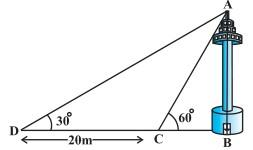# A TV tower stands vertically on a bank of a canal. From a point on the other bank directly opposite the tower, the angle of elevation of the top of the tower is 60°. From another point 20 m away from this point on the line joing this point to the foot of the tower, the angle of elevation of the top of the tower is 30° (see Fig.) . Find the height of the tower and the width of the canal.From diagaram

AB = tower = ?

CB = canal = ?

From right ΔABD,

tan 30° = AB/BD

1/√3 = AB/(20+BC)

AB = (20+BC)/√3 … (i)

Also,

In right ΔABC,

tan 60° = AB/BC

√3 = AB/BC

AB = √3 BC … (ii)

From equation (i) and (ii)

√3 BC = (20+BC)/√3

3 BC = 20 + BC

2 BC = 20

BC = 10

Substituting the value of BC in equation (ii)

AB = 10√3

This implies, the height of the tower is 10√3 m and the width of the canal is 10 m.(0)(0)